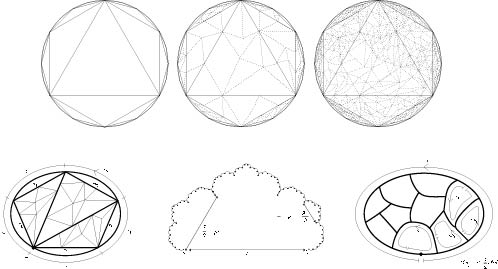Higher connectedness of asymptotic cones Tim R. Riley Topology, 42, pages 1289–1352, 2003 This article formed a large proportion of my doctoral thesis. We give coarse geometric conditions for a metric space X to have N-connected asymptotic cones. These conditions are expressed in terms of certain filling functions which are defined recursively on dimension and concern maps to X from the 0-skeleta of combinatorial spheres and discs. We prove that the asymptotic cones of X are N-connected if and only if the k-dimensional filling functions are bounded for k=1, 2,..., N+1. We apply this criterion in the case where X is a finitely generated group with a word metric. This leads to information about groups with simply connected cones - in particular they have linear filling length. We prove that if all the asymptotic cones of the group are N-connected then it is of type FN+1 and we provide bounds on N-th order isoperimetric and isodiametric functions. Also we show that the asymptotic cones of a virtually polycyclic group are all contractible if and only if the group is virtually nilpotent. cones.pdf, 66 pages, 6 figures